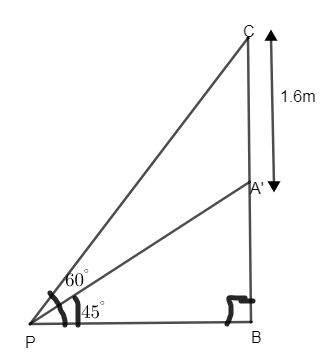QUESTION

# A statue 1.6m tall, stands on the top of a pedestal. From a point on the ground, the angle of elevation of the top of the statue is ${{60}^{\circ }}$ and from the same point the angle of elevation of the top of the pedestal is ${{45}^{\circ }}$. Find the height of the pedestal.

Hint: Draw the figure according to the question. We will get two triangles with angle ${{60}^{\circ }}$ and ${{45}^{\circ }}$. Thus use the basic trigonometric formula and find the height of the pedestal in which the statue stands.

Let the total height of the monument be BC. The height of the pedestal can be taken as AB. The length of the statue can be taken as AC, we have been given the length as 1.6m.$\therefore$ Length of statue, AC = 1.6m
The length of elevation from the ground to the top of the statue = ${{60}^{\circ }}$.
Thus from the figure we can say that, $\angle CPB={{60}^{\circ }}$.
Similarly, the angle of elevation from the ground to top of the pedestal is ${{45}^{\circ }}$. Thus from the figure we can say that, $\angle APB={{45}^{\circ }}$.

We need to find the height of pedestal i.e. length of AB.
The statue is perpendicular to the ground. So, $\angle PBC={{90}^{\circ }}$.
Now let us first consider, $\Delta APB$.
$\tan {{45}^{\circ }}$ = opposite side/ adjacent side $=\dfrac{AB}{PB}$

From the trigonometric table, $\tan {{45}^{\circ }}=1$.
\begin{align} & \therefore 1=\dfrac{AB}{PB} \\ & \Rightarrow AB=PB-(1) \\ \end{align}
Now let us consider, $\Delta CBP$.
$\tan {{60}^{\circ }}$ = opposite side/ adjacent side $=\dfrac{BC}{BP}$

From the trigonometric table, $\tan {{60}^{\circ }}=\sqrt{3}$.
$\sqrt{3}=\dfrac{BC}{BP}$, we know that BP = AB.
From figure, BC = BA + AC = BA + 1.6.

Substitute all these values we get,
\begin{align} & \sqrt{3}=\dfrac{AB+1.6}{AB} \\ & \sqrt{3}AB=AB+1.6 \\ & \Rightarrow \sqrt{3}AB-AB=1.6 \\ & AB\left( \sqrt{3}-1 \right)=1.6 \\ & \therefore AB=\dfrac{1.6}{\left( \sqrt{3}-1 \right)} \\ \end{align}

Let us rationalize the length of AB.
Multiply by $\left( \sqrt{3}+1 \right)$ in numerator and denominator.
$AB=\dfrac{1.6\left( \sqrt{3}+1 \right)}{\left( \sqrt{3}-1 \right)\left( \sqrt{3}+1 \right)}$ $\left\{ \because \left( a-b \right)\left( a+b \right)={{a}^{2}}-{{b}^{2}} \right\}$
\begin{align} & AB=\dfrac{1.6\left( \sqrt{3}+1 \right)}{{{\left( \sqrt{3} \right)}^{2}}-{{1}^{2}}}=\dfrac{1.6\left( \sqrt{3}+1 \right)}{3-1} \\ & AB=\dfrac{1.6\left( \sqrt{3}+1 \right)}{2}=0.8\left( \sqrt{3}+1 \right) \\ \end{align}

Thus we got the height of the pedestal as $0.8\left( \sqrt{3}+1 \right)$ m.

Note: Here Pedestal means the base or support on which a statue or column is mounted. Here angle of elevation from ground is given, so we can directly find the relations connecting the length of Pedestal. Don’t forget to rationalize to find the answer you get.